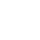CurrentHome>Resources
Phase Measurement of S3602 Vector Network Analyzer
Release time :2021.03.24 Reading quantity:63

Saluki S3602 series vector network analyzer has multiple calibration modes such as frequency response, single port, response isolation, enhanced response, double ports and electrical calibration, and multiple display modes such as the logarithmic amplitude, linearity amplitude, standing wave, phase, group delay and Smith chart. It is equipped with various standard interfaces, such as USB, LAN, GPIB and VGA.

This section introduces the basic knowledge of phase measurement.

1. Introduction of Phase Measurement

It is important to understand the amplitude and phase information of the tested device to integrate high-level devices. Similar to the amplitude measurement, the S-parameter is also applied in phase measurement. Phase measurement is a kind of relative (ratio) measurement instead of absolute measurement. The phase of the signal into the device (incident signal) and the response signal are compared in phase measurement. The response signal may be reflected signal or transmitted signal. Given that the analyzer is accurately calibrated, the phase difference (i.e. phase shift) of two signals is the measurement result of phase characteristics of the tested device. The following figure shows the phase offset between the incident signal and transmitted signal observed through the Oscilloscope.Fig.1 Phase shift between signals

2. Reason to Perform Phase Measurement

Phase measurement is an important function of the vector network analyzer. Fig.2 shows the reasons for accurate measurement of the amplitude and phase.Fig.2 Reasons for accurate amplitude and phase measurement

If the element and circuit are used to transmit signals in the communication system, signal distortion must be within the normal limits. The signal distortion can be divided into two types.

1) Linear distortion: refer to the failure to keep the flat amplitude and linear phase shift within the relevant frequency range as a result of frequency changes.

2) Nonlinear distortion: refer to the new spectrum component of the circuit, such as AM-PM transformation.

It is important to accurately measure the amplitude and phase characteristics of the element or circuit, so as to ensure the efficient transmission or adsorption of energy in the circuit and prevent the distortion in signal transmission. A good example to prevent distortion is to measure the complex impedance of the antenna.

3. Use of Phase Format of Analyzer

The phase format of the analyzer is used to display the phase changes over the frequency or power. The phase difference beyond±180° between the reference signal and response signal cannot be measured by the analyzer. If the phase value varies from +180° to -180°, the sawtooth type phase measurement track will be displayed on the analyzer. The sawtooth waveform may not reach +180° or -180° sometimes in measurement at the discrete frequency points. The data points of +180° and -180° may not be the points of sweep measurement.Fig.3 Phase format of analyzer

4. Type of Phase Measurement

1) Complex impedance: the complex impedance data such as the resistance, reactance, amplitude and phase can be determined by S11 and S22 measurement and observed in the Smith chart and polar coordinate format.

2) AM-PM transformation: the unexpected phase offset may be caused by system amplitude changes in the AM-PM transformation measurement, defined as the output phase change of each 1dB increase of the power input into the amplifier, in degree/dB. Measurement is done at the 1dB gain compression point.

3) Linear phase offset: refer to the phase distortion produced in measurement of the tested device. Ideally, the phase shift of the tested device is linear to the frequency. The deviation produced relative to the theoretical phase offset is referred to as the linear phase offset (also known as the phase linearity).

4) Group delay: it is another way to measure the phase distortion of the device. The transition time of the signal of specific frequency through the device is measured, and the group delay is calculated according to the derivative of the measured phase response.

5. Linear Phase Offset and Group Delay

The linear phase offset and group delay are phase information of the measurement device but for different purposes.

1) Advantages of linear phase offset measurement:

a) The noise is lower than that of group delay measurement.

b) The characteristics of the device transmitting phase-modulated signals can be better represented. In this case, it is more appropriate to use the phase as the unit instead of the second.

2) Advantages of group delay measurement:

a) Compared with the linear phase offset measurement, phase distortion can be explained more easily.

b) The characteristics of the tested device can be represented accurately. The phase ripple slope is calculated by the analyzer in group delay measurement and depends on the ripple quantity within the unit frequency. Phase responses with the same phase ripple peak-peak value are compared. Large phase slope response may result in large group delay changes and signal distortion.